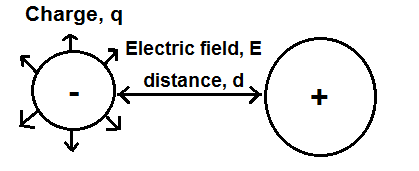﻿ Electric Potential Energy Calculator ﻿# Electric Potential Energy CalculatorCharge (q): C Electric Field (E) N/C Distance (d) m

0.00J

This electric potential energy calculator calculates the electric potential energy of an object based on the object's charge, q, the electric field, E, of the object, and the distance, d, between the charged object we are measuring the electric potential energy of against another charge to which we are comparing it, according to the formula shown above. Electric potential energy is the amount of energy which a charged object has before it commences motion; in other words, it's the potential energy that a charged object has.

To use this calculator, a user just enters the charge, q, of the object, measured in coulombs (C), the electric field, E, of the charged object, measured in meters per second (N/C), and the distance, d, of the charged object to another charged object to which it is being compared to, measured in meters (m). The result of the computation is in unit joules (J).

An electric potential energy calculator can be useful for electronics, especially when viewing it from a more physics perspective, because it can be used to calculate the potential energy of electrons that aren't in motion. While electrons are just gathered together and are not moving, they are in a static state, and this is called static electricity. The opposite of this is when electrons are in motion, which is called kinetic energy. See Kinetic Energy Calculator to calculate kinetic energy. To calculate the amount of energy that electrons have while not moving, all we have to do is calculate the electric potential energy.

Related Resources

﻿